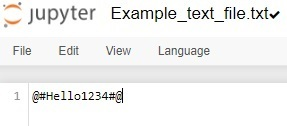# 使用正则表达式统计Python程序中大写字母、小写字母、特殊字符和数字值的个数

• 大写字母 –
• 模式 – [A-Z]

• 解释 – 模式[A-Z]匹配从A到Z的任何大写字母。连字符-指定了一个字符的范围。因此，此模式可以匹配输入字符串中的任何大写字母。

• 小写字母 –

• 模式 – [a-z]

• 解释 – 模式[a-z]匹配从a到z的任何小写字母。与大写字母类似，此模式捕获输入字符串中的任何小写字母。

• 特殊字符 –

• 模式 – [^A-Za-z0-9]

• 解释 – 模式[^A-Za-z0-9]匹配任何不是大写字母、小写字母或数字的字符。方括号[^ ]中的插入符号^表示否定。因此，此模式匹配范围不在A-Z、a-z或0-9之内的任何特殊字符。

• 数字值 –

• 模式 – [0-9]

• 解释 – 模式匹配从0到9的任何数字。

## 输入输出情况

Input string: Hello World!
Output:
Uppercase letters: 2
Lowercase letters: 8
Special characters: 1
Numeric values: 0


Input string: @#Hello1234#@
Output:
Uppercase letters: 1
Lowercase letters: 4
Special characters: 4
Numeric values: 4


### 例

import re

def count_characters(input_string):
uppercase_count = len(re.findall(r'[A-Z]', input_string))
lowercase_count = len(re.findall(r'[a-z]', input_string))
special_count = len(re.findall(r'[^A-Za-z0-9]', input_string))
numeric_count = len(re.findall(r'[0-9]', input_string))

return uppercase_count, lowercase_count, special_count, numeric_count

# define the input string
input_str = 'Tutor1als!p0int'
upper, lower, special, numeric = count_characters(input_str)

print("Uppercase letters:", upper)
print("Lowercase letters:", lower)
print("Special characters:", special)
print("Numeric values:", numeric)


### 输出

Uppercase letters: 1
Lowercase letters: 11
Special characters: 1
Numeric values: 2


### 示例import re

def count_characters(filename):
with open(filename, 'r') as file:
for line in file:
uppercase_count = len(re.findall(r'[A-Z]', line))
lowercase_count = len(re.findall(r'[a-z]', line))
special_count = len(re.findall(r'[^A-Za-z0-9]', line))
numeric_count = len(re.findall(r'[0-9]', line))

return uppercase_count, lowercase_count, special_count, numeric_count

# Provide the path of the text file
file = 'Example_text_file.txt'

# Call the function to count Uppercase, Lowercase, special character and numeric values
upper, lower, special, numeric = count_characters(file)

print("Uppercase letters:", upper)
print("Lowercase letters:", lower)
print("Special characters:", special)
print("Numeric values:", numeric)


### 输出

Uppercase letters: 1
Lowercase letters: 4
Special characters: 4
Numeric values: 4


• 微信订阅
• 回顶